# GSEB Solutions Class 9 Maths Chapter 14 Statistics Ex 14.3

Gujarat Board GSEB Solutions Class 9 Maths Chapter 14 Statistics Ex 14.3 Textbook Questions and Answers.

## Gujarat Board Textbook Solutions Class 9 Maths Chapter 14 Statistics Ex 14.3

Question 1.
A survey conducted by an organization for the cause of illness and death among the women between the ages 15-44 (in years) worldwide, found the following figures (in %).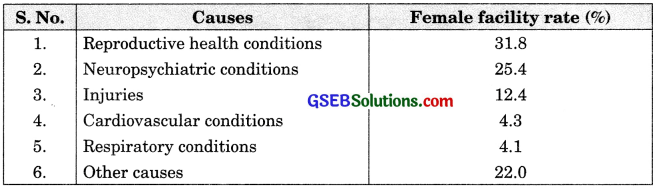Represent the information given above graphically.
Which condition is the major cause of women’s ill health and death worldwide?
Try to find out, with the help of your teacher, any two factors which play a major role in the cause in
(ii) above being the major cause.
Solution: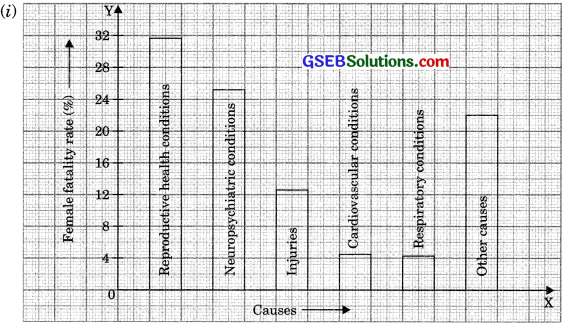(ii) Reproductive health conditions is the major cause of women’s health and death worldwide.
(iii) Lack of proper diet, lack of advised exercises.Question 2.
The following data on the number of girls (to the nearest ten) per thousand boys in different sections of the Indian society is given below:

 Section Number of girls per thousand boys Scheduled Caste (SC) 940 Scheduled Tribe (ST) 970 Non-SC/ST 920 Backward districts 950 Non-backward districts 920 Rural 930 Urban 910

Represent the information above by a bar graph.
In the classroom discuss what conclusions can be arrived at from the graph.
Solution: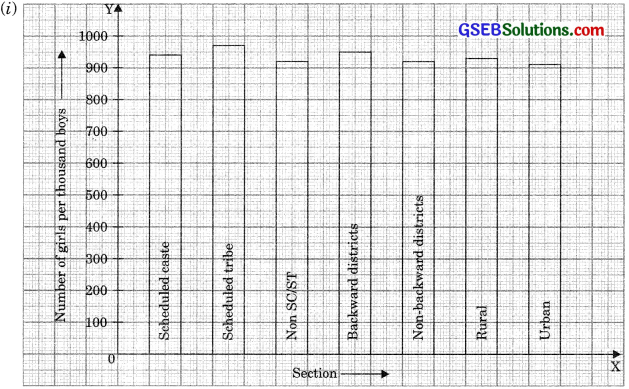(ii) The two conclusions we can arrive at from the graph are as follows:
(a) The number of girls to the nearest ten per thousand boys is maximum in the scheduled tribe section of the society and minimum in the urban section of the society.
(b) The number of girls to the nearest ten per thousand boys is the same for ‘Non-SC/ST’ and ‘Non-backward districts’ sections of the society.Question 3.
Given below are the seats won by different political parties in the polling outcome of state assembly elections:(i) Draw a bar graph to represent the polling results.
(ii) Which political party won the maximum number of seats?
Solution: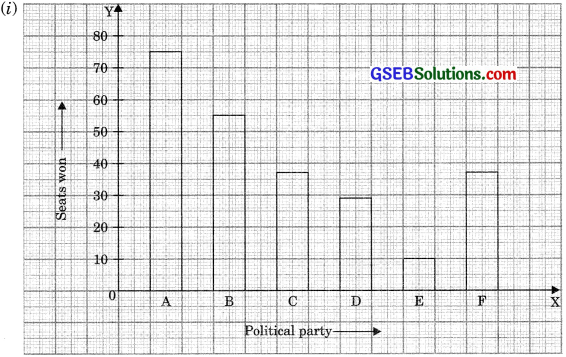(ii) Political party A won the maximum number of seatsQuestion 4.
The length of 40 leaves of a plant is measured correct to one millimeter, and the obtained data is represented in the following table:(i) Draw a histogram to represent the given data correct to one millimeter, and the obtained data is in the following represented table:
(ii) Is there any other suitable graphical representation for the same data?
(iii) Is it correct to conclude that the maximum number of leaves is 153 mm long? Why?
Solution:
(i) Modified continuous distribution is as follows(ii) Frequency polygon.
(iii) No, because the maximum number of leaves have their lengths lying in the interval 145-153. ‘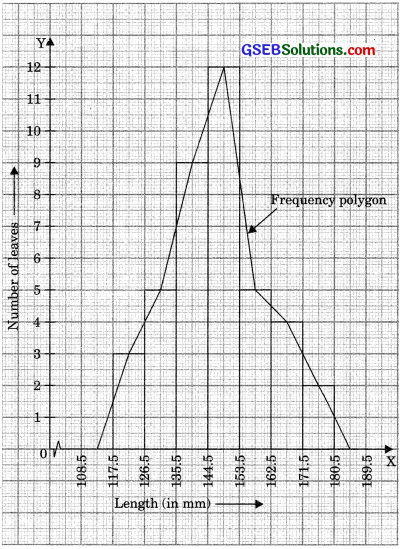Question 5.
The following table gives the lifetimes of 400 neon lamps:(i) Represent the given information with the help of a histogram,
(ii) How many lamps have a lifetime of more than 700 hours?
Solution:(ii) 74 + 62 + 48 = 184 lamps have a lifetime of more than 700 hours.Question 6.
The following table gives the distribution of students of two sections according to the marks obtained by them: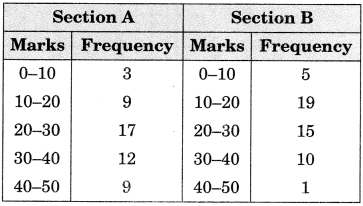Represent the marks of the students of both the sections on the same graph by two frequency polygons. From the two polygons compare the performance of the two sections.
Solution:
Modified tables:
For Section A:For Section B: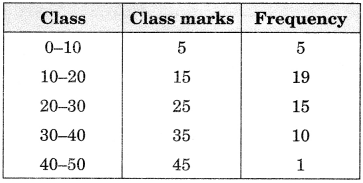From the graph, it can be observed that students. The performance of section A is better than section B in terms of good marks.Question 7.
The runs scored by two teams A and B on the first 60 balls in a cricket match are given below: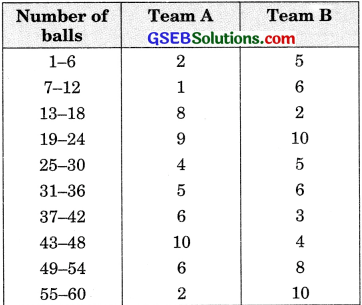Represent the data of both the teams on the same graph by frequency polygons.
(Hint: First make the class interval continuous).
Solution:
Modified table: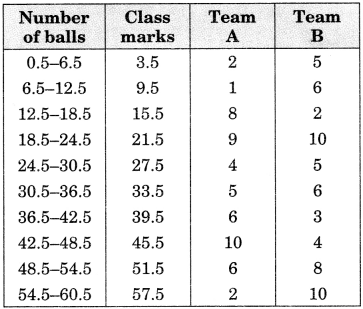Question 8.
A random survey of the number of children of various age groups playing in a park was found as follows: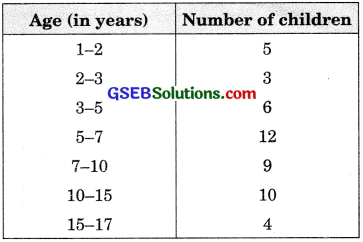Draw a histogram to represent the data above.
Solution:
Modified table
(Minimum class size = 1)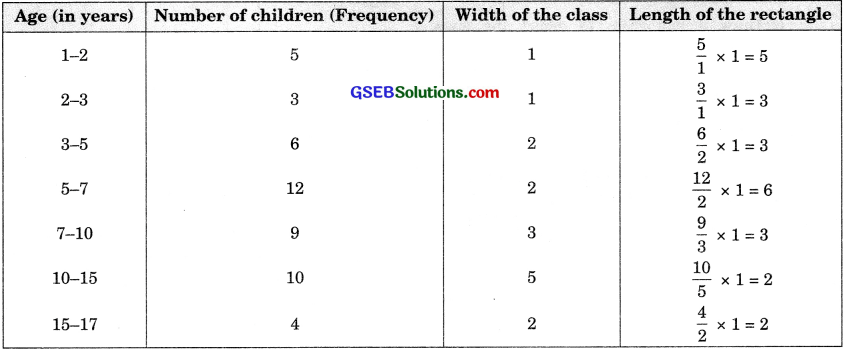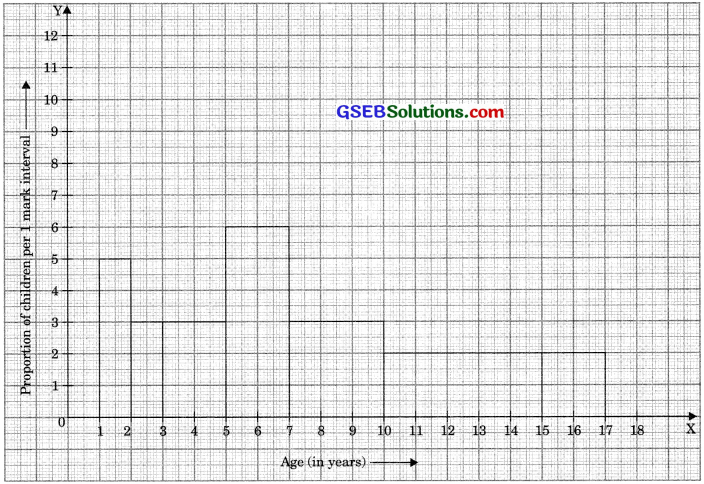Question 9.
100 surnames were randomly picked up from a local telephone directory and a frequency distribution of the number of letters in the English alphabet in the surnames was found as follows: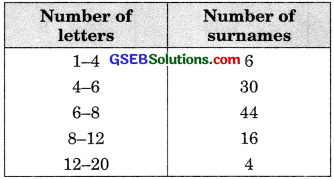(i) Draw a histogram to depict the given information.
(ii) Write the class interval in which the maximum number of surnames lies.
Solution:
(i) Modified table:
(Minimum class size = 2)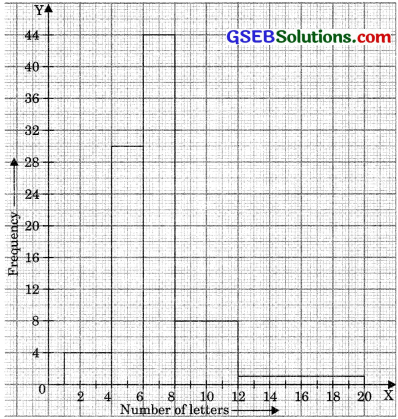(ii) The class interval in which the maximum number of surnames lie is 6-8.